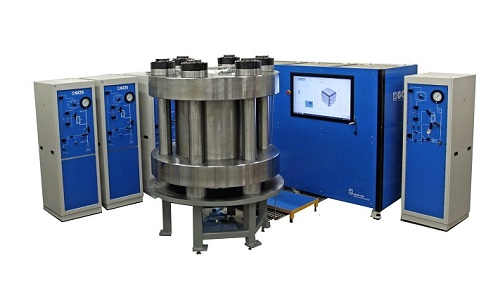Related ArticlesAn example of a true triaxial test system (Courtesy of GCTS)

# True Triaxial Test

## Overview

In a standard triaxial test, only two principal stresses are controlled—the axial stress (σ1) and the confining stress (σ3). The intermediate principal stress (σ2) is assumed to be equal to the confining pressure due to the geometry of the cylindrical test specimen. However, in the field, a soil element experiences different stresses in all three orthogonal directions. Sometimes, these stresses are very significant. In order to replicate the field conditions more accurately, the true triaxial test was developed. In a true triaxial test, all three principal stresses are independently controlled.An example of a soil true triaxial testing system

(Courtesy of GCTS)

## How is a True Triaxial Test Performed?

A true triaxial test is performed very similarly to the Soil Triaxial Test and the Rock Triaxial Test. However, these tests make use of a confining liquid to apply pressure to the specimen. In the true triaxial test, six pistons are used to apply a load in each of the three directions. Some systems use only four pistons (placed symetrically) to apply loads and use a confining pressure to apply load in the final orthogonal direction. This allows the user to apply, remove, or keep the load constant in all three orthogonal directions. Some triaxial systems have sliding plates on the pistons, which allow all the plates to move simultaneously during deformation of the soil or rock specimen without any of the pistons exerting a load on another piston while still applying the load to the entire face of the specimen.

## What does a True Triaxial Test Specimen Look Like?

A true triaxial specimen is either cubical or cuboidal in shape. Typically this is done with a mold and a membrane for soil specimen, like in the Soil Triaxial Test, or with a saw and grinder for a rock specimen. Different systems are capable of testing specimen of many different sizes, and some systems can test specimen up to 12 in. by 12 in. by 24 in.

## How are Soil and Rock Characteristics Determined?

The angle of internal friction and cohesion of a specimen are determined through an analysis of the Mohr’s Circle outputs for multiple specimens. This is done with the same method as described for the Soil Triaxial Test.

## What are the Trade-Offs of a True Triaxial Test?

The true triaxial test gives the user much more control over the different stress paths, or ways to get from one set of stresses to another, the specimen can follow. However, this means that the systems needed to perform this test are much larger, more complex, and more expensive than a simple triaxial test. By using pistons to act on each side of the specimen, the sliding faces can produce friction on each other and on the specimen, which will alter data significantly without proper testing equipment. The pistons also do not cause stresses on the corners of the specimen, which can lead to non-uniform stress distributions within the specimen.

Keywords: Rock Polyaxial Test — Rock Polyaxial Testing System — Rock True Triaxial Test — Compression Test — True Triaxial Testing Machine — Polyaxial Testing Instrument — Rock Triaxial Test — True Triaxial Test — Polyaxial Test — Shear Strength — Mohr’s Circle